Geometry

# Similar Triangles Problem Solving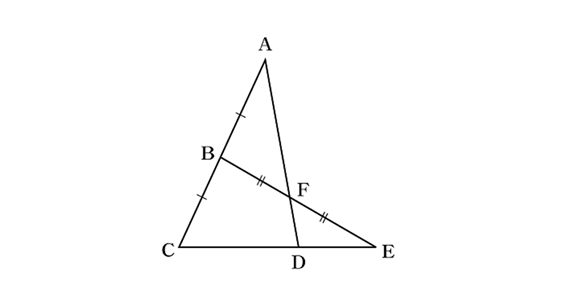Let $\lvert\overline {AB}\rvert$ denote the length of $\overline {AB}.$ Then in the above diagram, $\lvert\overline {AB}\rvert = \lvert\overline {BC}\rvert$ and $\lvert\overline {BF}\rvert = \lvert\overline {FE}\rvert.$ If $\lvert\overline{CD}\rvert=38,$ what is $\lvert\overline {DE}\rvert?$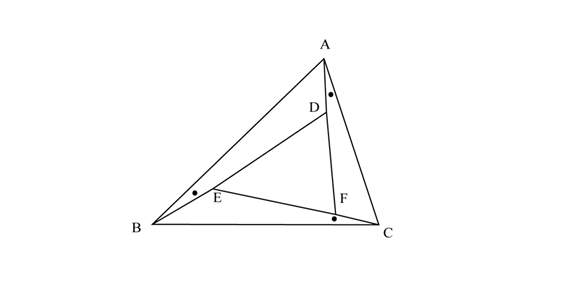In the above diagram, $\angle ABD = \angle BCE = \angle CAF.$ Given the lengths $\lvert \overline{AB}\rvert=12, \lvert \overline{AC}\rvert =7, \lvert \overline{BC}\rvert = 14,$ what is $\lvert \overline{DE}\rvert : \lvert \overline{EF}\rvert : \lvert \overline{DF}\rvert?$

Note: The above diagram is not drawn to scale.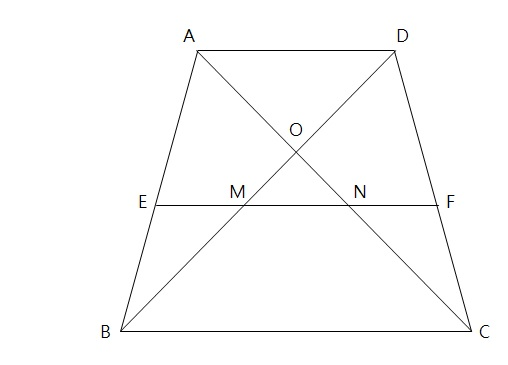In the above quadrilateral $ABCD,$ $\overline{AD} \parallel \overline{EF} \parallel \overline{BC},$ and

$\lvert\overline{AE}\rvert = 2\lvert\overline{EB}\rvert, \lvert\overline{BM}\rvert = 4, \lvert\overline{AD}\rvert = 6, \lvert\overline{BC}\rvert = 9,$ where $\lvert\overline{AE}\rvert$ denotes the length of $\overline{AE}.$ What is $\lvert\overline{DO}\rvert?$

Note: The above diagram is not drawn to scale.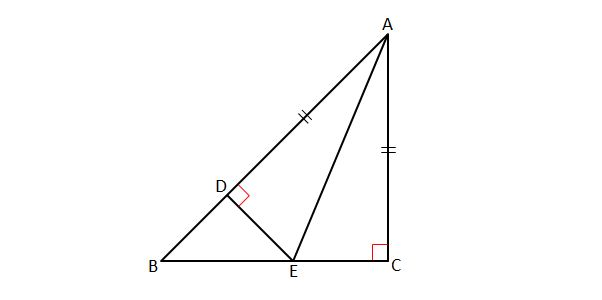In the above diagram, $\triangle ABC$ is a right-angled triangle where $\angle C$ is a right angle and $\lvert{\overline{AC}}\rvert=\lvert{\overline{BC}}\rvert.$ If $\lvert{\overline{AC}}\rvert=\lvert{\overline{AD}}\rvert,$ $\overline{AB} \perp \overline{DE}$ and $\lvert{\overline{CE}}\rvert=7,$ what is the measure of $\overline{DB} ?$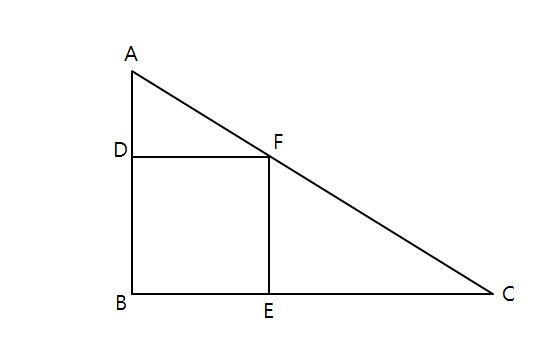$\triangle ABC$ is a right triangle with side lengths $\lvert\overline{AB}\rvert = 25, \lvert\overline{BC}\rvert = 50$ If $\square{DBEF}$ in the above diagram is a square, what is the area of $\square{DBEF}?$

×